# Standard Effects Analysis in Within-Subject Study Designs with SPSS and Python

Posted on
by Mo Kari

On a related note, for questionnaire analysis check-out my repo under https://github.com/MohamedKari/questionnaire-analysis.

# Problem Setup

“The purpose of a repeated measures designs is to determine the effect of different experimental conditions on a dependent variable” (Rutherford 2001, p. 61) from measures taken on the same subject under these different conditions.

Let’s assume we follow a within-subject design, measuring the dependent variable “Duration” (or the mean of Duration across multiple repetitions) across 7 different participants under the the independent variables “Distance” and “Size”. Distance has 3 levels: “Far”, “Mid”, “Near”. Size has 2 levels: “Big”, “Small”. This results in `3x2=6` different conditions.

Let’s assume our trial data is in a table of the following form:

``````ParticipantId,Distance,Size ,Duration
1            ,near    ,small,2060.770833333333
1            ,near    ,big  ,2816.020833333333
1            ,far     ,small,4552.916666666667
1            ,far     ,big  ,5382.895833333333
1            ,mid     ,small,1847.0625
1            ,mid     ,big  ,2052.3125
2            ,near    ,small,1802.5208333333333
2            ,near    ,big  ,2169.0
2            ,far     ,small,2743.2291666666665
2            ,far     ,big  ,2159.1041666666665
2            ,mid     ,small,1923.56
2            ,mid     ,big  ,1749.42
3            ,near    ,small,1635.8333333333333
3            ,near    ,big  ,2204.785714285714
3            ,far     ,small,3470.673076923077
3            ,far     ,big  ,2937.096153846154
3            ,mid     ,small,1876.0238095238096
3            ,mid     ,big  ,1846.071428571429
``````

The table should have 7 participants x 6 conditions = 42 rows. It should have 4 columns: 1 column for participant ID, 1 column for the depedendent variable and one column for each independent variable.

# Tooling

## Running a Multi-Factor ANOVA in SPSS

### Data Preparation

In order to run the analysis in SPSS, we need to create a table in Wide-Column Format, where each of the 6 conditions is represented by a column, each of the subjects is represented by a row, and the measurement of the dependent variable is given in the resulting cells:

``````ParticipantId,mid_small         ,mid_big           ,near_small        ,near_big          ,far_small         ,far_big           ,reach_small       ,reach_big
1            ,1847.0625         ,2052.3125         ,2060.7708333333335,2816.0208333333335,4552.916666666667 ,5382.895833333333 ,3583.0            ,3509.9375
6            ,1756.6666666666667,1798.3333333333333,1610.3095238095239,2299.404761904762 ,2845.609756097561 ,2878.829268292683 ,2092.5714285714284,2827.714285714286
``````

The table should have 7 participants = 7 rows, and 6 conditions + 1 column for the participant id = 7 columns.

### Workflow in SPSS

Given such a table, we can run the ANOVA-RM in SPSS as follows: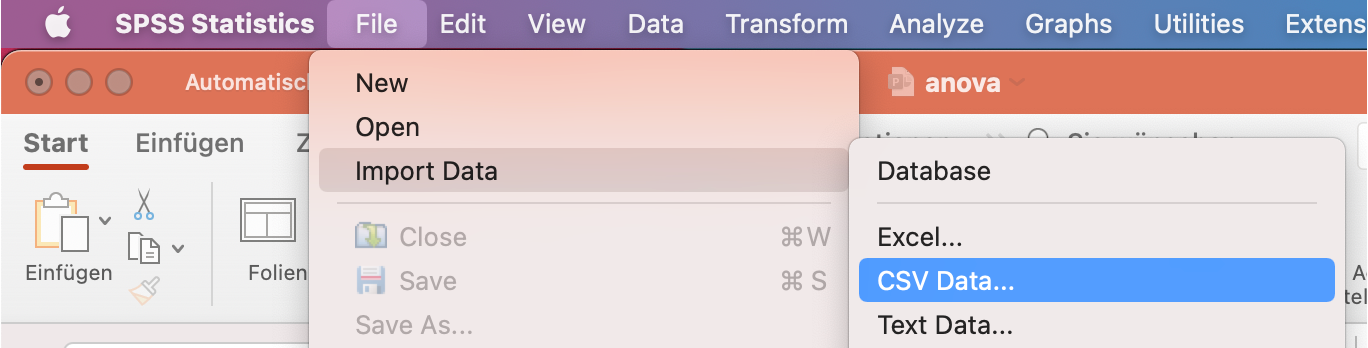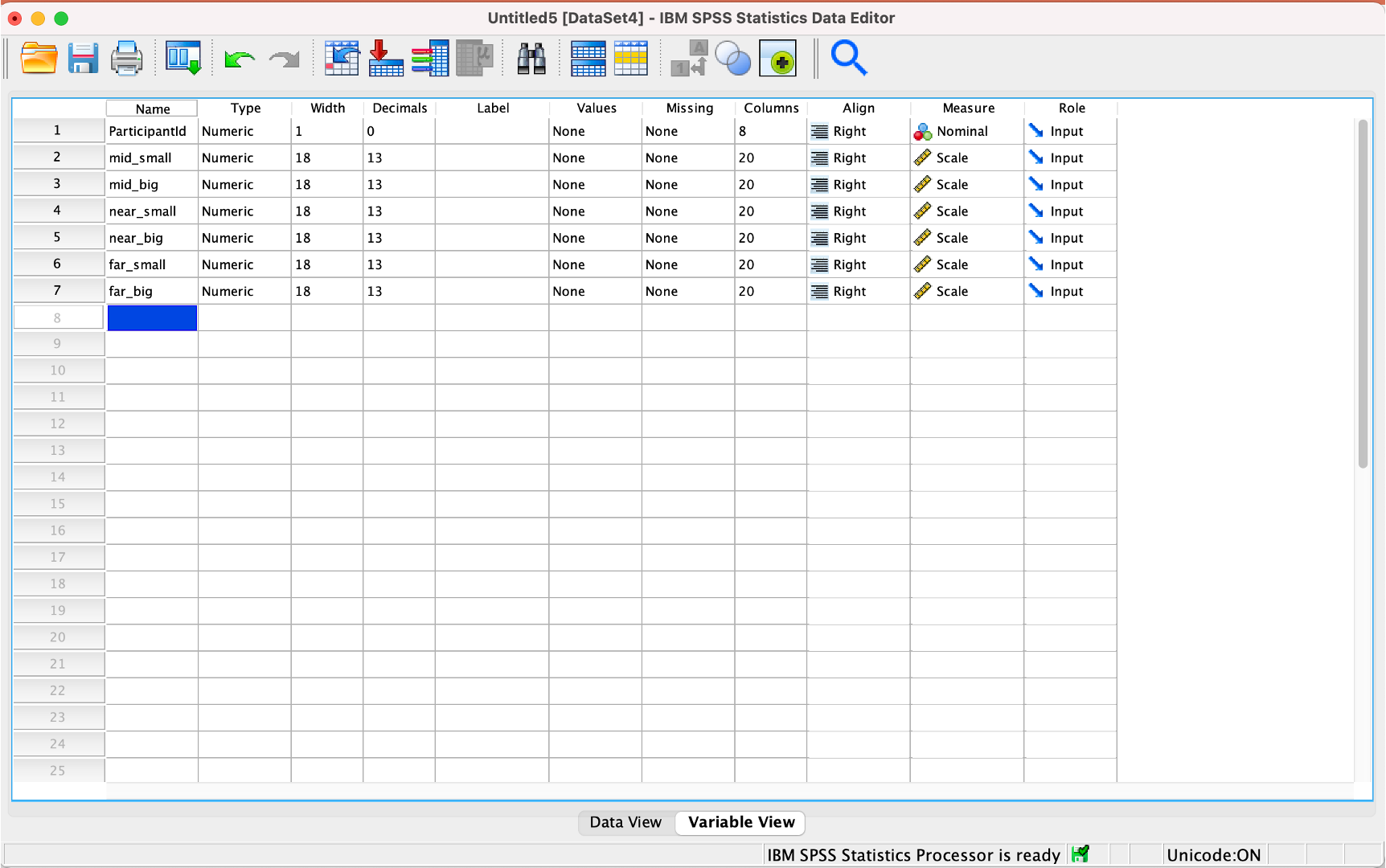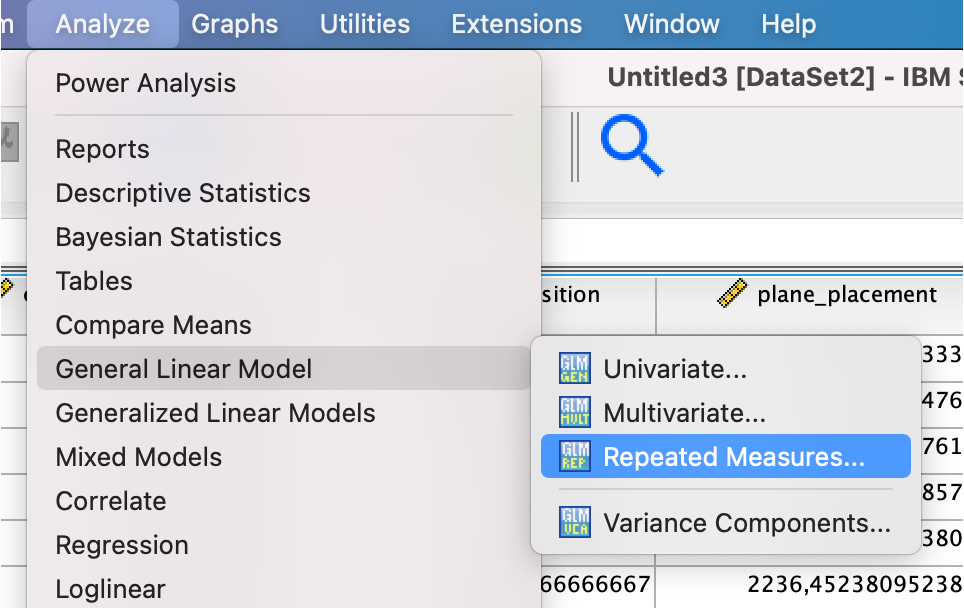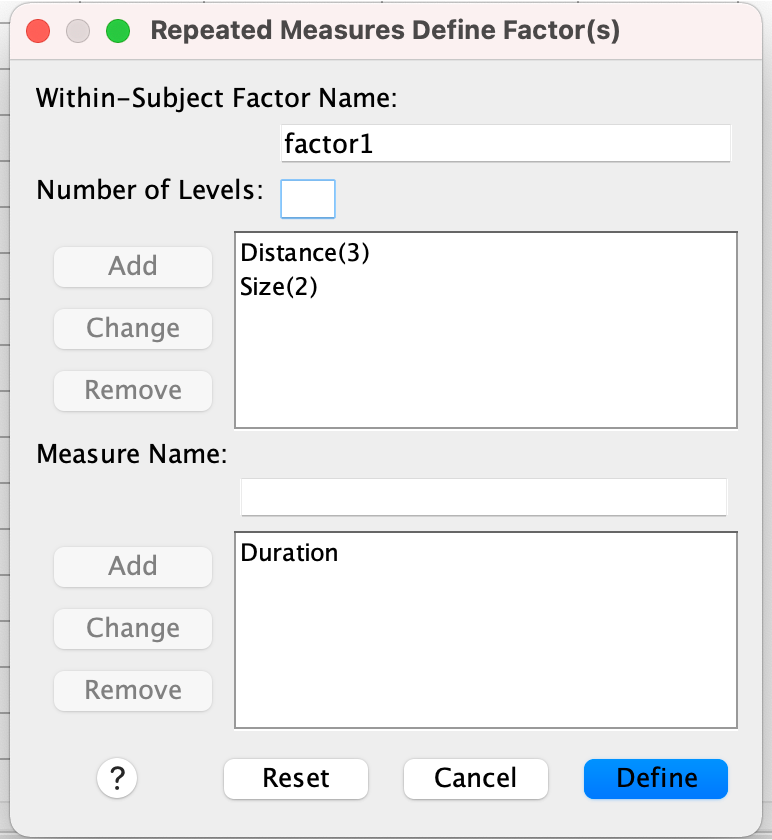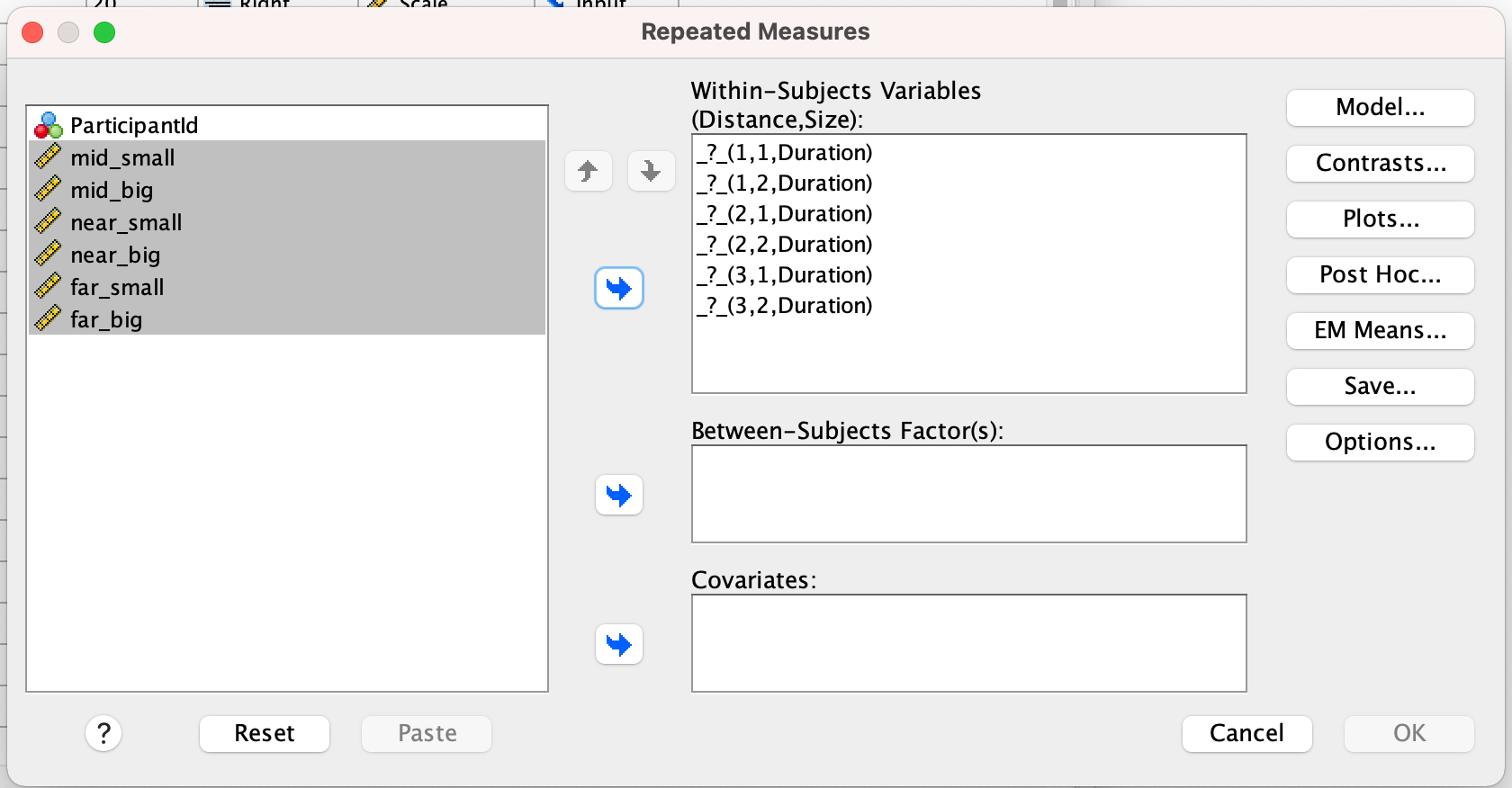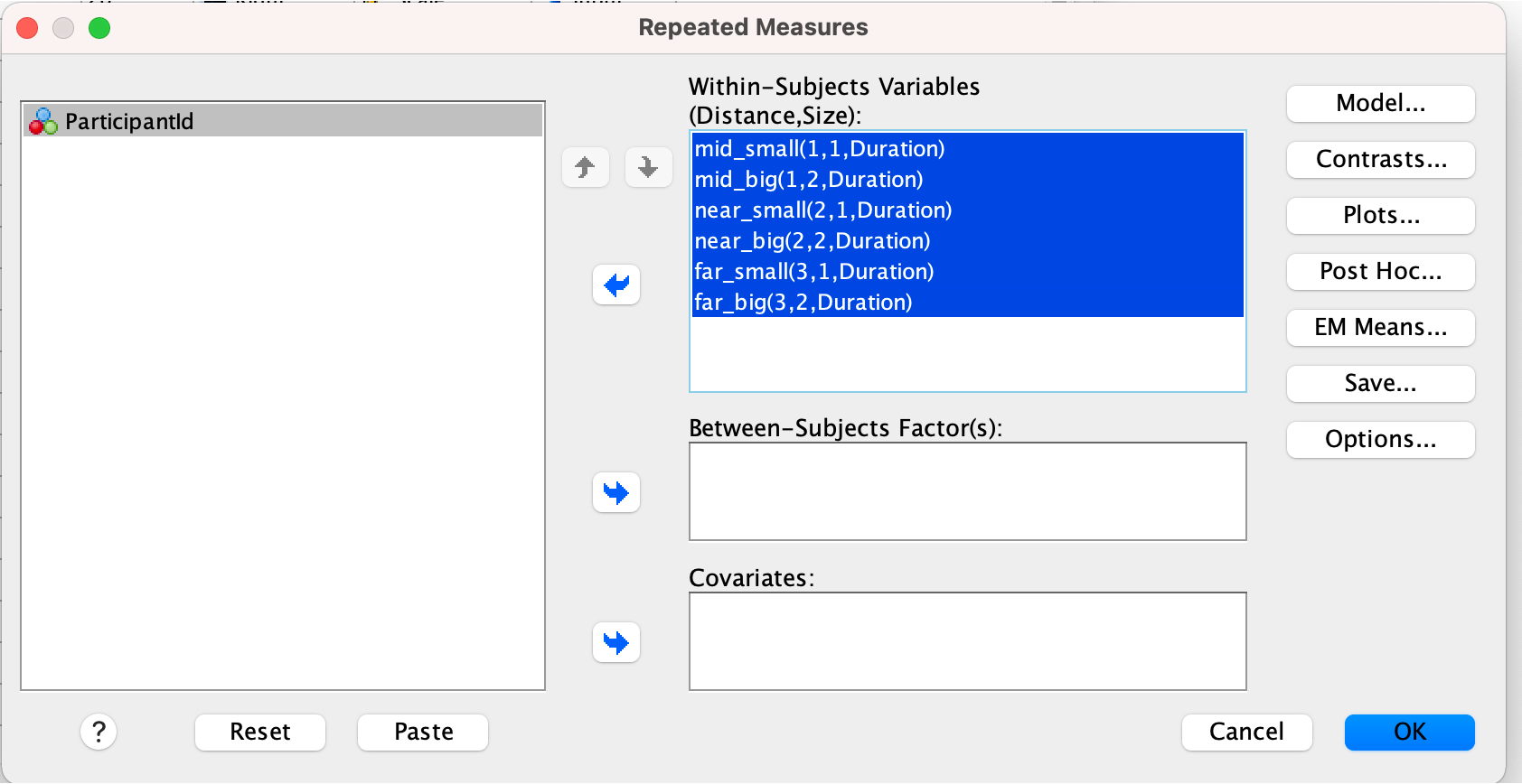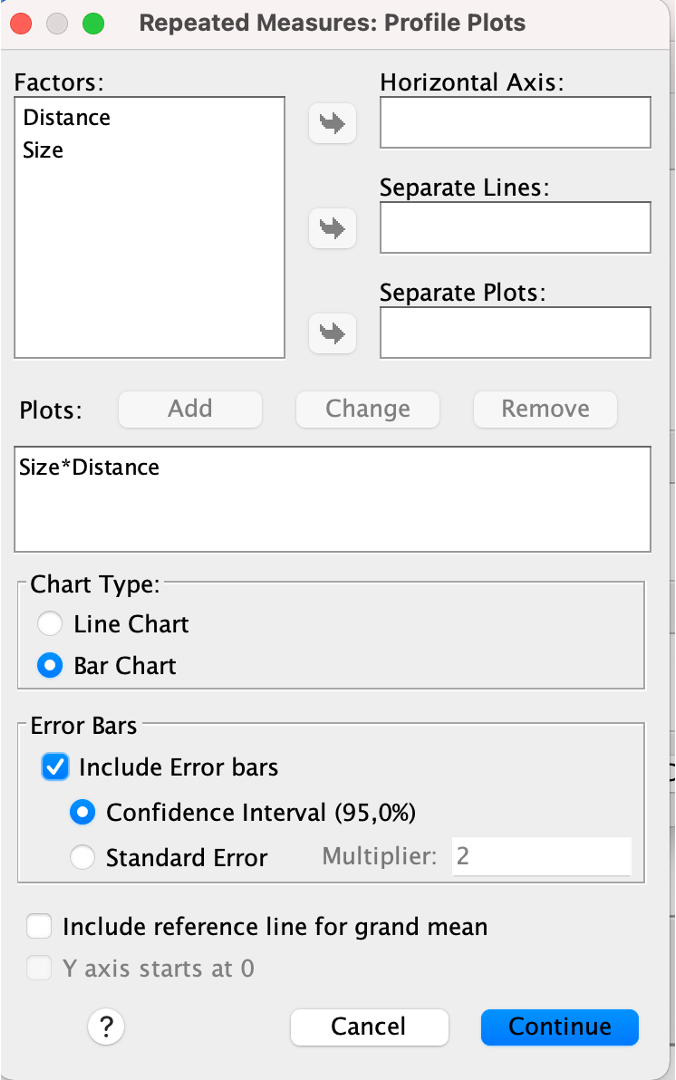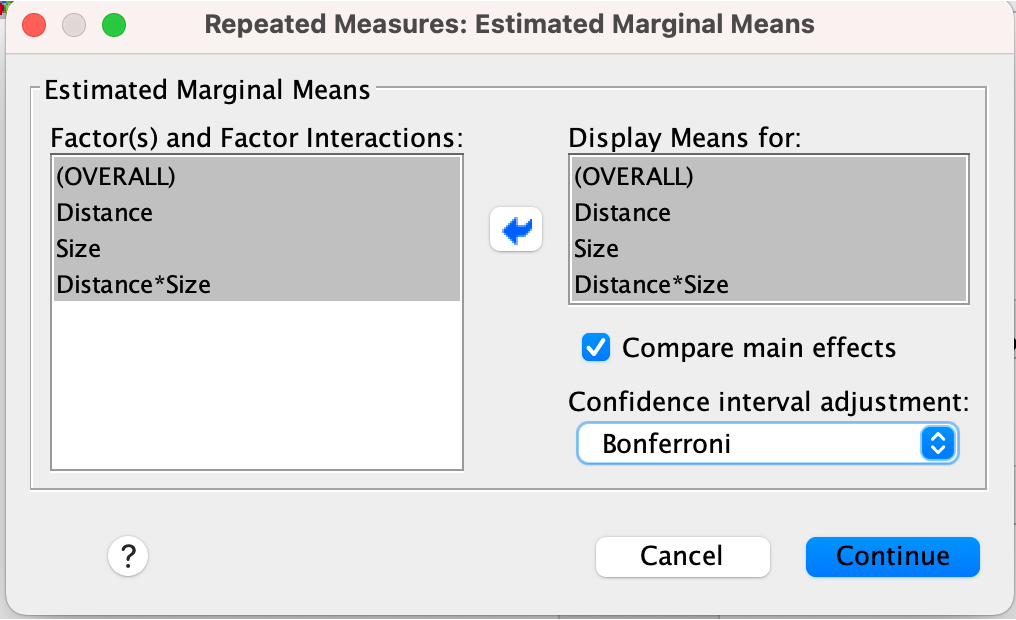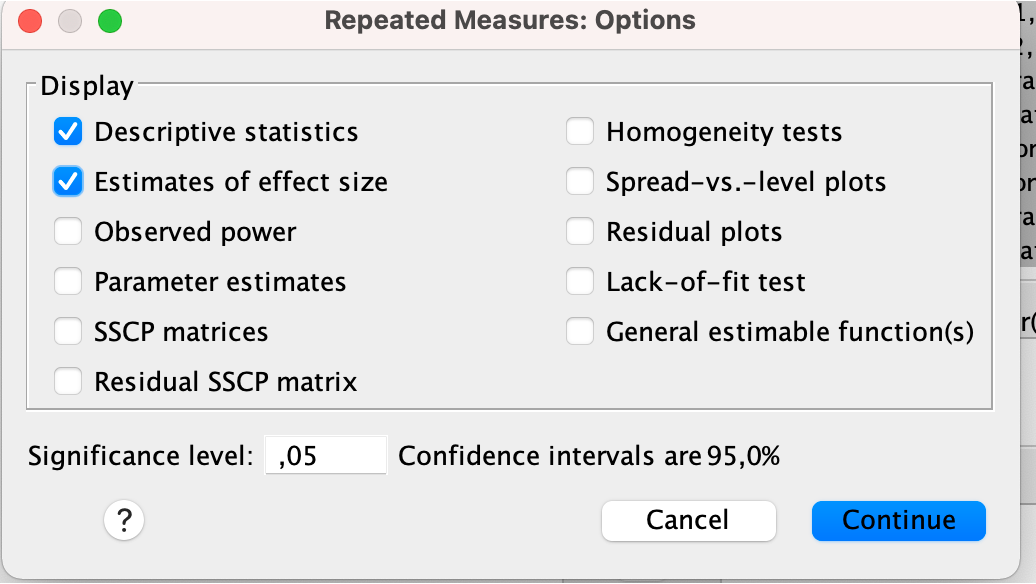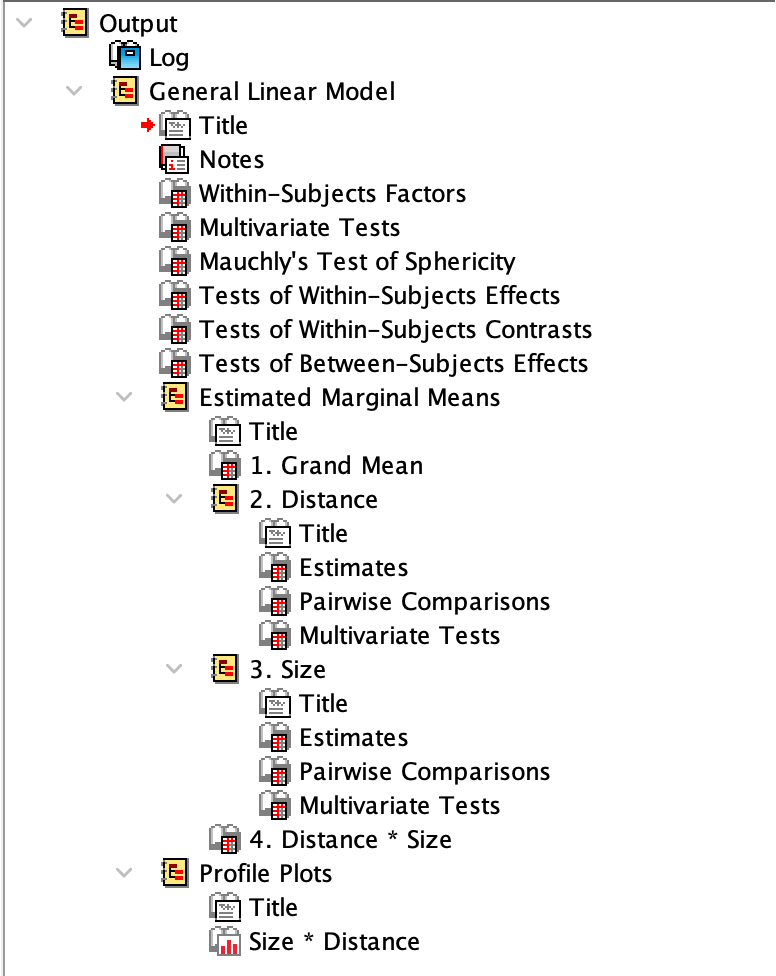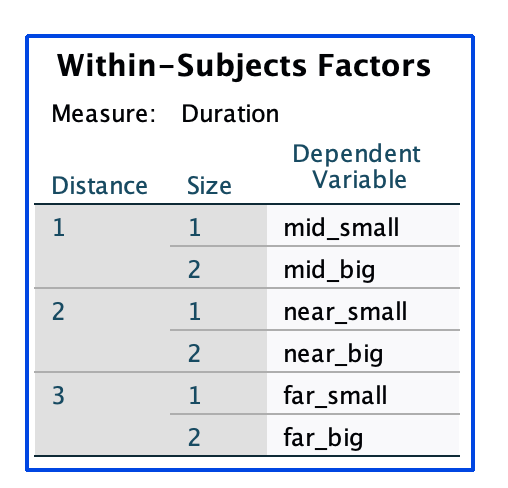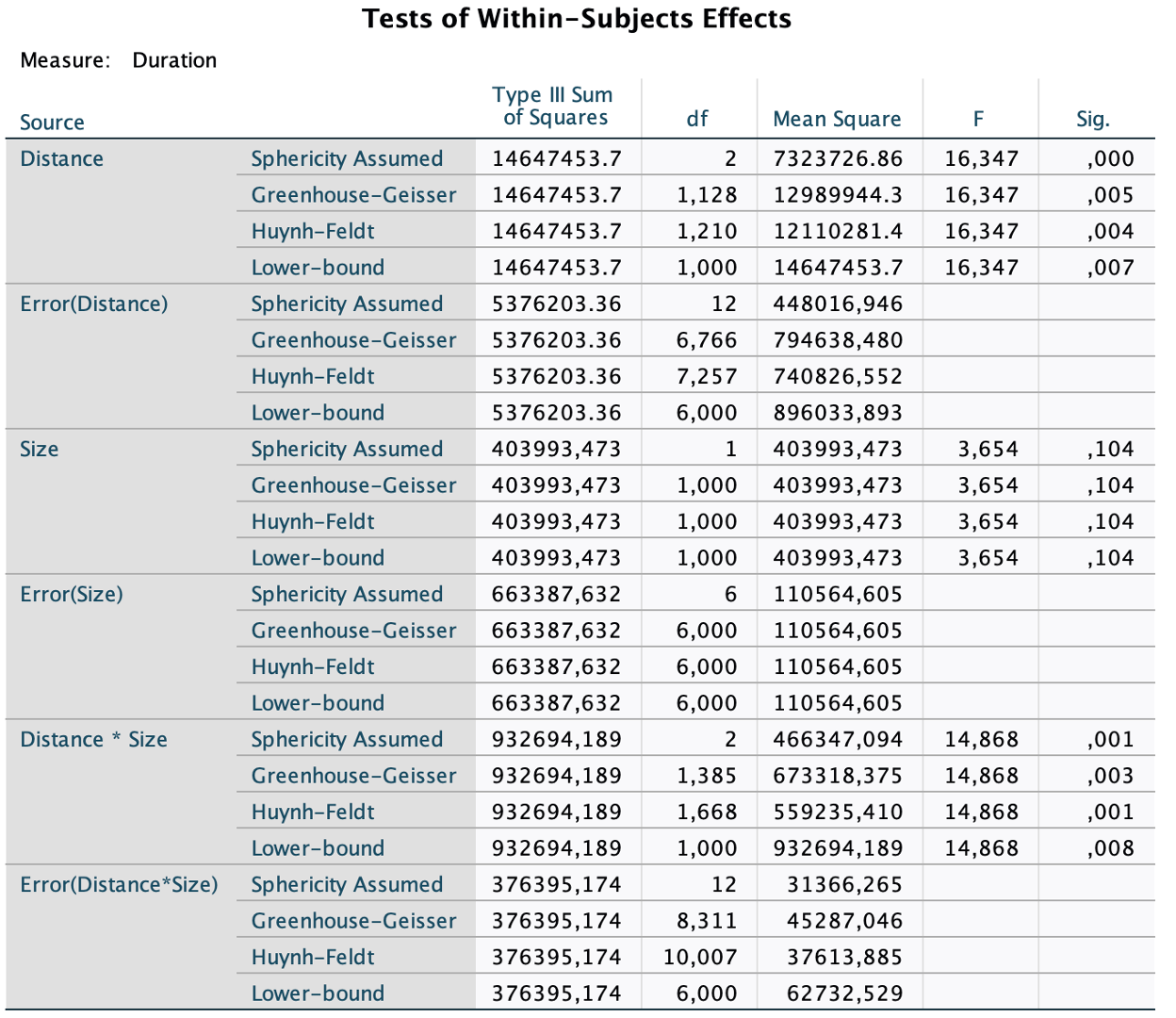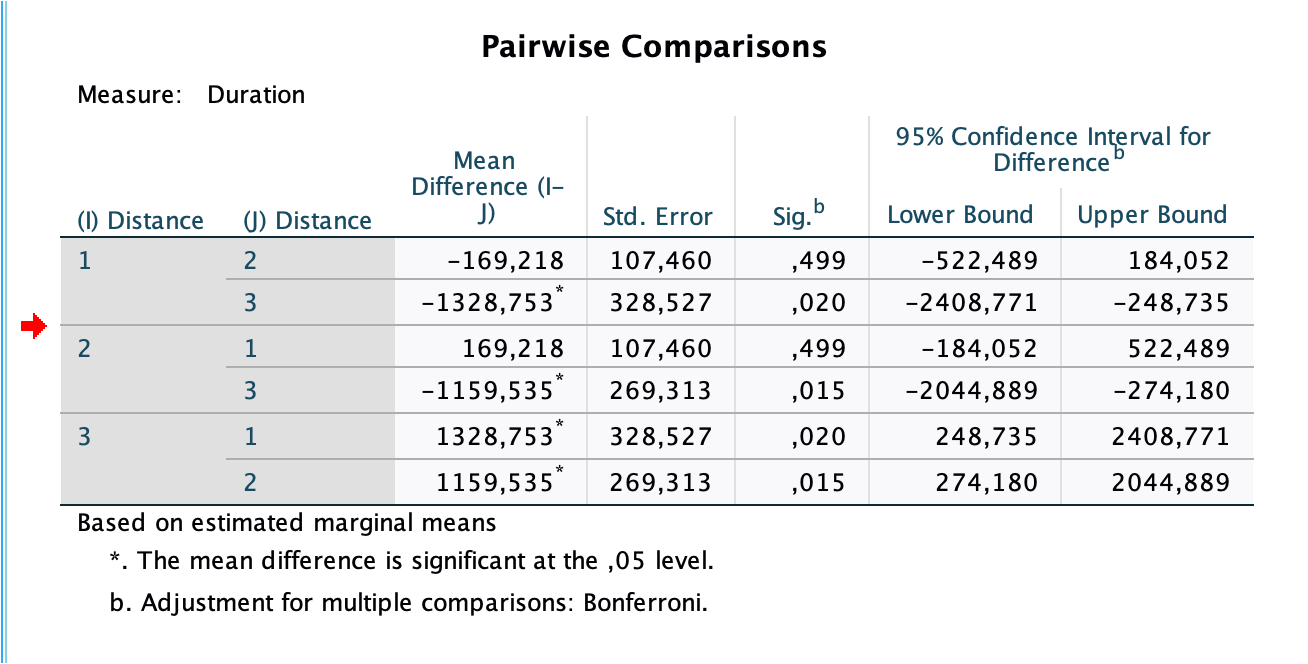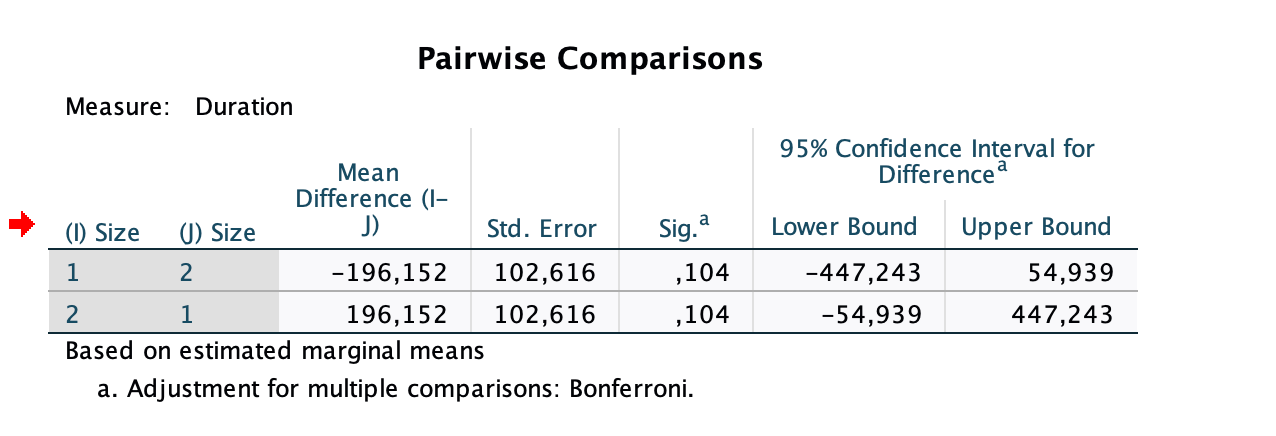### Interpretation

In the within-subject effects, we report the F-value with an index, composited from the the degrees of freedom n-1, where n is the number of factors, and the degrees of freedom in the error term. The degrees of freedom in the error term are generally given by the number of observations, decremented by the number of degrees of freedorm (i. e. number of levels minus 1). As numbers of observations, we consider the degrees of freedom per subject, i. e.:

• dof_model = dof_1 = number of levels - 1
• dof_error = dof_2 = (dof_1 * n_participants) - dof_1

Then, we report F_(dof_model, dof_error) as the F-value.

## Running a Multi-Factor ANOVA in Python

SciPy, StatsModels, scikit-posthoc all offer certain capabilities to run a repeated-measured ANOVA, however I have found pingouin to offer the richest output while still only requiring one-liners:

With pingouin, we achieve the same results as in SPSS with the following script:

``````import pandas as pd
import pingouin as pg
from pingouin import pairwise_ttests

def run():

anova_results = pg.rm_anova(data=df, dv="Duration", subject="ParticipantId", within=["Distance", "Size"], detailed=True)
pairwise_results = pairwise_ttests(dv='Duration', within="Distance", subject='ParticipantId', data=df, padjust="bonf")

print(anova_results)
print(pairwise_results)

if __name__ == "__main__":
run()
``````
``````➜  within-subject-anova git:(main) ✗ python3 anovarm.py
Source            SS  ddof1  ddof2            MS          F     p-unc  p-GG-corr       np2      eps
0         Distance  1.464745e+07      2     12  7.323727e+06  16.346986  0.000375   0.004655  0.731507  0.56380
1             Size  4.039935e+05      1      6  4.039935e+05   3.653913  0.104487   0.104487  0.378490  1.00000
2  Distance * Size  9.326942e+05      2     12  4.663471e+05  14.867792  0.000565   0.002935  0.712476  0.69261
Contrast    A     B  Paired  Parametric         T  dof alternative     p-unc    p-corr p-adjust    BF10    hedges
0  Distance  far   mid    True        True  4.044577  6.0   two-sided  0.006768  0.020303     bonf   9.087  1.810697
1  Distance  far  near    True        True  4.305526  6.0   two-sided  0.005062  0.015186     bonf  11.393  1.574290
2  Distance  mid  near    True        True -1.574709  6.0   two-sided  0.166391  0.499173     bonf   0.843 -0.543070
``````